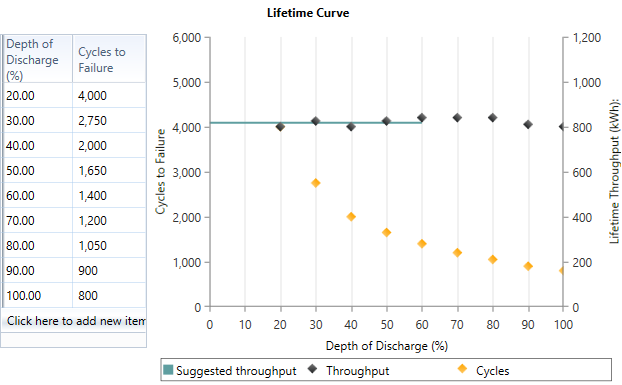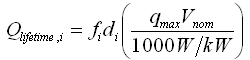# HOMER Pro 3.15

 Navigation: Library > Components Library > Storage Library Idealized ModelThe Idealized Storage Model replicates a simple storage model that assumes a flat discharge curve because the supply voltage stays mostly constant during the discharge cycle. For this model, you need to enter only the nominal capacity in amp-hours. HOMER uses this as the actual capacity of the storage. The Idealized Storage Model works well for some high-performance Lithium Ion batteries.

To create a new storage component that uses the Idealized Storage Model, start by copying a component that uses it, such as the Generic 1kWh Li-Ion battery. Change the variables for the new component.

## General

 Variable Description Nominal Voltage The rated voltage. It is called nominal because the actual voltage varies according to the storage unit's operating conditions and state of charge. This input is used to convert specifications in A or Ah to values in kW or kWh. Nominal Capacity The rated capacity in amp hours. It is the total capacity of the storage system. Round Trip Efficiency The round-trip, DC-to-storage-to-DC efficiency of the storage bank. HOMER assumes that the percentage loss on charge and on discharge are the same. Minimum State of Charge The relative state of charge below which the storage bank is never drawn. Maximum Charge Rate The maximum allowable charge rate of the storage component, measured in amps per amp-hour of unfilled capacity. Maximum Charge Current The absolute maximum charge current, in amps. Maximum Discharge Current The absolute maximum discharge current, in amps.

For the Battery lifetime is limited by option, select the time, throughput, or time and throughput radio button. If you select:

Time, the storage unit requires replacement after a fixed length of time (float life, years).

Throughput, the storage unit requires replacement after a fixed quantity of energy cycles through it (throughput, kWh).

Time and Throughput, the storage unit requires replacement when the first of these two values is reached.

If you choose to limit the storage life to a certain number of years, enter that number of years as the Float life. If you choose to limit the storage life to a certain quantity of energy throughput, enter that quantity as the Lifetime throughput. You can also check the Enter lifetime curve box to help calculate this lifetime throughput value.

In a lifetime test, the tester subjects the storage unit to repeated regular charge and discharge cycles. Each cycle, the storage is discharged to a certain depth, then fully charged again. The lifetime test determines how many such cycles the storage unit can withstand before it needs to be replaced. Manufacturers perform a series of these tests at different depths of discharge to create the storage unit's lifetime curve and determine the longevity of their products. A lifetime curve shows the number of cycles to failure versus the depth of the cycles, as shown below.You specify the storage lifetime curve in HOMER as a table of cycles to failure versus depth of discharge. HOMER plots that series of points as yellow diamonds. As in the example above, that plot typically shows a sharp decrease in the number of cycles to failure with increasing depth of discharge. HOMER also plots the lifetime throughput, which it calculates for each point in the lifetime curve using the following equation:where: Qlifetime,i = the lifetime throughput [kWh] fi = the number of cycles to failure di = the depth of discharge [%] qmax = the maximum capacity of the storage [Ah] Vnom = the nominal voltage of the storage [V]

HOMER plots these values as black diamonds on the lifetime curve (using the right-hand y-axis). Their values typically show only a weak dependence on the depth of discharge. HOMER's simulation logic makes the simplifying assumption that the lifetime throughput does not depend on the depth of discharge. The horizontal black line in the lifetime curve shows the calculated value of lifetime throughput. The line is the average of the throughput values calculated for all the points you enter. It is only drawn across the allowable range of depth of discharge.

The calculated lifetime throughput is for reference only; HOMER uses the Lifetime throughput (kWh) input near the top of the page in the simulation. If you want to use the calculated throughput value, be sure to copy it to the Lifetime throughput (kWh) input.